# Logical operations and boolean functions

Logic operations also known as Boolean functions, part of Boolean algebra, are widely used in computer science, engineering and mathematics. There are different words and expression used for them, like logic gates or bitwise operations, but the main principle is the same: performing logical operations on bits (`0` and `1` values).

Electronics is now part of nearly every engineering domain, therefore it’s very important that engineers have a minimum understanding of logic, bitwise operations.

Most of the physical calculations are performed with decimal numbers. This is because we use decimal numbers for all the physical values (e.g. 10 A, 250 Nm, 120 km, etc.). Computers use binary numbers to perform calculations. To recall how to convert a decimal number in a binary number, read the article Decimal to Binary Conversion.

In parallel with arithmetic operations (addition, subtraction, multiplication, division), there are also logic operations. These are used to evaluate if a logical expression is either `true` or `false`.

In our examples we are going to use two symbols A and B, called inputs. Each one of them can have either a `true` value (`1`) or a `false` value (`0`). After a logic operations is going to be performed on the inputs, we are going to obtain a result, with the symbol Q, called output. Similar with the inputs, the output Q can only have a `true` (`1`) or a `false` (`0`) value.

The logical state/value `true`, also called `HIGH`, is equivalent with the binary value `1`. The logical value `false`, also called `LOW`, is equivalent with the binary value `0`.

 `true` `HIGH` `1` `false` `LOW` `0`

The most common logic operations (also called gates, operators) are:

• NOT
• AND
• OR
• NAND
• NOR
• XOR
• XNOR

Each operation has a symbol assigned (block diagram) and a truth table. The symbol is used for designing graphical diagrams of logical operations. There are different standards for the symbols, the most common being ANSI (American National Standards Institute) and IEC (International Electrotechnical Commission).

The truth table is defining how the logic (logical) operation works, what is the value of the output Q, function of the value of the inputs A and B.

### NOT gate

The logic operation NOT is also called an inverter or negation, because it is inverting the boolean value of the input. For example, if A is `true`, applying a NOT operation to it will give the result Q as `false`. In the same way, if A is `false`, applying a NOT gate to it will give the result Q as `true`.

 Logic gate ANSI symbol IEC symbol Truth table NOT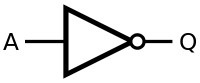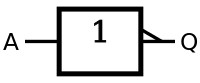input output A Q=NOT A 0 1 1 0

### AND gate

The logic operation AND will return a `true` value only if both inputs have a `true` value. Otherwise, if one or both inputs contain a `false` value, the AND gate will output a `false` value. We can say that the AND gate effectively finds the minimum between two binary inputs.

 Logic gate ANSI symbol IEC symbol Truth table AND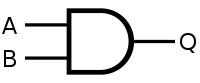input output A B Q=A AND B 0 0 0 0 1 0 1 0 0 1 1 1

### OR gate

The logic operation OR will return a `true` value if at least one of the inputs has a `true` value, and a `false` value if none of the inputs has a `true` value. We can say that the OR gate effectively finds the maximum between two binary inputs.

 Logic gate ANSI symbol IEC symbol Truth table OR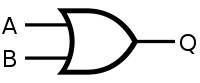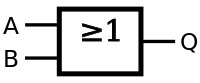input output A B Q=A OR B 0 0 0 0 1 1 1 0 1 1 1 1

### NAND gate

The logic operation NAND gate (negative/not AND) produces a `false` output only if all its inputs are `true`. The NAND gate can be regarded as a complement of the AND gate. If one or both inputs are `false`, the NAND gate outputs a `true` result.

 Logic gate ANSI symbol IEC symbol Truth table NAND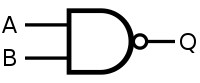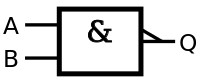input output A B Q=A NAND B 0 0 1 0 1 1 1 0 1 1 1 0

### NOR gate

The logic operation NOR (negative/not OR) produces a `true` output only when both inputs are `false`, otherwise produces a `false` output. With other words, if only one or both inputs are `true`, the NOR operator outputs a `false` result. The NOR gate is the result of the negation of the OR operator.

 Logic gate ANSI symbol IEC symbol Truth table NOR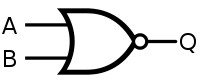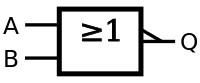input output A B Q=A NOR B 0 0 1 0 1 0 1 0 0 1 1 0

### XOR gate

The XOR logical operator (pronounced exclusive OR) gives a `true` output only when the inputs have different states. If the inputs have the same logical states, both either `true` or `false`, the XOR gate outputs a `false` result. To output a `true` result, only one of the inputs has to be `true`, the other one must be `false`.

 Logic gate ANSI symbol IEC symbol Truth table XOR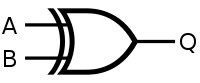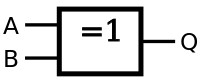input output A B Q=A XOR B 0 0 0 0 1 1 1 0 1 1 1 0

### XNOR gate

The XNOR logical operator (pronounced exclusive NOR) is the logical complement of the XOR gate. A `true` output is result if the inputs have the same logical state (either both `true` or both `false`). If the inputs have different logical values, the XNOR gate outputs a `false` result.

 Logic gate ANSI symbol IEC symbol Truth table XNOR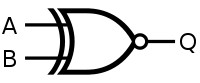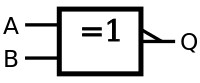input output A B Q=A XNOR B 0 0 1 0 1 0 1 0 0 1 1 1

All the above logic operators (gates) are summarized in the table below.

 A B AND OR NAND NOR XOR XNOR 0 0 0 0 1 1 0 1 0 1 0 1 1 0 1 0 1 0 0 1 1 0 1 0 1 1 1 1 0 0 0 1

For any questions or observations regarding this tutorial please use the comment form below.

Don’t forget to Like, Share and Subscribe!

#### Ad Blocker Detected

Dear user, Our website provides free and high quality content by displaying ads to our visitors. Please support us by disabling your Ad blocker for our site. Thank you!